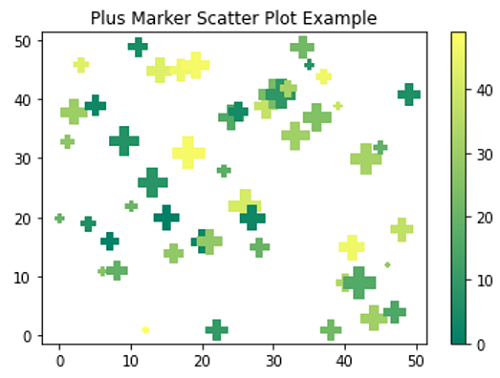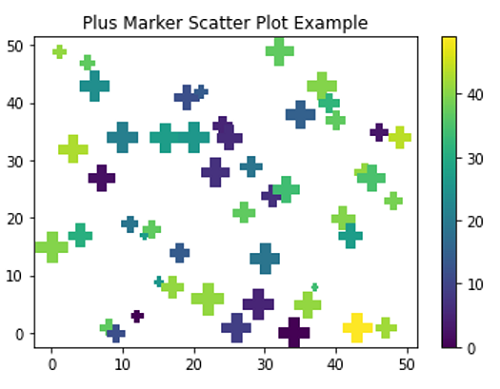# Python | Plus (+) Scatter Marker

In this tutorial, we are going to learn how to use plus (+) shape scatter marker in scatter plot using matplotlib in Python?
Submitted by Anuj Singh, on August 17, 2020

There are few markers that can be used everywhere such as circular or square markers. But, matplotlib has some other inbuilt defined markers such as plus(+) shape marker which is used in marking plots. In matplotlib.pyplot command marker='4' for our desired marker style and the following figure illustrates the example of the same.

Illustrations:## Python code for plus (+) scatter marker

```import numpy as np
import matplotlib.pyplot as plt

# Example 1
x = np.arange(50)
y = np.random.randint(0,50,50)
ss = np.random.randint(0,50,50)
c = np.random.randint(0,50,50)

plt.figure()
plt.scatter(x,y, s=ss*10, c=c, marker='P', cmap='summer')
plt.title('Plus Marker Scatter Plot Example')
plt.colorbar()

# Example 2
x = np.arange(50)
y = np.random.randint(0,50,50)
ss = np.random.randint(0,50,50)
c = np.random.randint(0,50,50)

plt.figure()
plt.scatter(x,y, s=ss*10, c=c, marker='P')
plt.title('Plus Marker Scatter Plot Example')
plt.colorbar()

# Example 3
x = np.arange(50)
y = np.random.randint(0,50,50)
ss = np.random.randint(0,50,50)
c = np.random.randint(0,50,50)

plt.figure()
plt.scatter(x,y, s=ss*10, c=c, marker='P', cmap='GnBu')
plt.title('Plus Marker Scatter Plot Example')
plt.colorbar()
```

Output:

```Output is as Figure
```